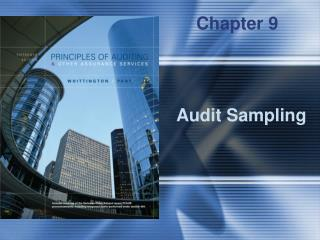DownloadDownload PresentationAudit Sampling

# Audit Sampling

Télécharger la présentation## Audit Sampling

- - - - - - - - - - - - - - - - - - - - - - - - - - - E N D - - - - - - - - - - - - - - - - - - - - - - - - - - -
##### Presentation Transcript

1. Chapter 9 Audit Sampling

2. Applying a procedure to less than 100% of a population To estimate some characteristic of the population Qualitative Quantitative What is Audit Sampling?

3. Design efficient samples Measure sufficiency of evidence Objectively evaluate sample results Advantages of Statistical Sampling

4. Random number tables Random number generators Systematic selection Selection of Random Sample

5. Random Number Table

6. Attributes sampling Discovery sampling Classical variables sampling Probability-proportional-to-size sampling Types of Statistical Sampling Plans

7. When planning the sample consider: The relationship of the sample to the relevant audit objective Materiality or the maximum tolerable misstatement or deviation rate Allowable sampling risk Characteristics of the population Select sample items in such a manner that they can be expected to be representative of the population Sample results should be projected to the population Items that cannot be audited should be treated as misstatements or deviations in evaluating the sample results Nature and cause of misstatements or deviations should be evaluated Requirements of AuditSampling Plans

8. True State of Population Deviation Rate Deviation Rate Exceeds Is Less Than Auditors’ Conclusion Tolerable Rate Tolerable Rate From the Sample Is: Deviation Rate Exceeds Tolerable Rate Deviation Rate Is Less Than Tolerable Rate Sampling Risks--Tests of Controls Correct Decision Incorrect Decision (Risk of Assessing Control Risk Too High) Incorrect Decision (Risk of Assessing Control Risk Too Low) Correct Decision

9. Determine the objective of the test Define the attributes and deviation conditions Define the population to be sampled Choose an audit sampling technique Specify: The risk of assessing control risk too low The tolerable deviation rate Estimate the population deviation rate Determine the sample size Select the sample Test the sample items Evaluate the sample results Document the sampling procedure Audit Sampling for Tests of Controls

10. True State of Population Misstatement in Misstatement in Account Exceeds Account Is Less Auditors’ Conclusion Tolerable Amount Than Tolerable From the Sample Is: Amount Misstatement in Account Exceeds Tolerable Amount Misstatement in Account Is Less Than Tolerable Amount Sampling Risks--Substantive Tests Correct Decision Incorrect Decision (Risk of Incorrect Rejection) Incorrect Decision (Risk of Incorrect Acceptance) Correct Decision

11. Determine the objective of the test Define the population and sampling unit Choose an audit sampling technique Determine the sample size Select the sample Test the sample items Evaluate the sample results Document the sampling procedure Audit Sampling for Substantive Tests

12. ItemPopulation APopulation B 1 2,100 8,000 2 2,100 25 3 2,100 2,000 4 2,100 400 5 2,100 75 Mean 2,100 2,100 Standard deviation -0- 3,395 The variability determines how much information each of the items in the population tells you about the other items in the population. Population Variability—Why it Matters

13. Factors Affecting Sample Size

14. Sample size = Determining Sample Size--MPU

15. Determining Sample Size--MPU Sampling Planned allowance for sampling risk Tolerable misstatement ______________________ = Incorrect acceptance coef. 1 + Incorrect rejection coef.

16. Adjusted allowance for sampling risk = Tolerable_ (Population size * Incorrect acceptance coef. * Sample stan. dev.) misstatementSample size This formula “adjusts” the allowance for sampling risk to consider the standard deviation of the audited values in the sample. It holds the risk of incorrect acceptance at its planned level. Variables Sampling Illustration--MPU

17. Variables Sampling Illustration--MPU Using the text example with a standard deviation of audited values of \$16 Adjusted allowance for sampling risk = Tolerable _ (Population size * Incorrect acceptance coef. * Sample stan. dev.) misstatement Sample size = \$364,000 _ (\$100,000 * 1.64 * \$16) 225 = \$189,067 • We would still “accept” the book balance because the \$6,250,000 (book value) falls within this interval Estimate of total + Adjusted allowance audited value for sampling risk \$6,100,000 + \$189,067 [\$5,910,933 to \$6,289,067]

18. Acceptance Interval

19. Plan Sample: Population: Size = 363 items Book value = \$200,000 Tolerable misstatement = \$10,000 Risk assessments: Inherent and control risk = Slightly below maximum Other substantive tests = Moderate Nonstatistical Variables Sampling Illustration

20. Sample size = Population book value X Reliability factor Tolerable misstatement = \$200,000 X 2.0 = 40 items \$10,000 Nonstatistical Sampling--Determination of Sample Size

21. Sample results: 40 accounts in sample \$350 net overstatement \$60,000 book value of sample items Projected misstatement: = [Sample net misstatement] X Book value of population [ Book value of sample ] = [ \$350 ] X \$200,000 [\$60,000] = \$1,167 Since the projected misstatement is only 11.7 percent (\$1,167/\$10,000) of tolerable misstatement, it is likely that the auditors would conclude that the account balance is materially correct. Nonstatistical Sampling--Evaluation of Sample Results

22. Population book value = \$6,250,000 Other Information: Tolerable misstatement = \$364,000 Sampling risk--Incorrect acceptance = 5% Expected misstatement = \$50,000 Use Figures 9-14 and 9-15 to obtain a “reliability factor” and an “expansion factor.” PPS Sampling Illustration

23. Sample size = Recorded amount of population * Reliability factor Tolerable misstatement - (Expected misstatement * Expansion factor) = \$6,250,000 * 3.0 = 66 \$364,000 - (\$50,000 * 1.6) Sampling interval = Book value of the population Sample size = \$6,250,000 = \$95,000 (approximately) 66 PPS Sample Size Computation

24. Upper Limit on misstatement = Projected misstatement + Basic precision (Rel. factor x interval) + Incremental allowance PPS Evaluation of Results

25. Calculation of Upper Limit on Misstatement